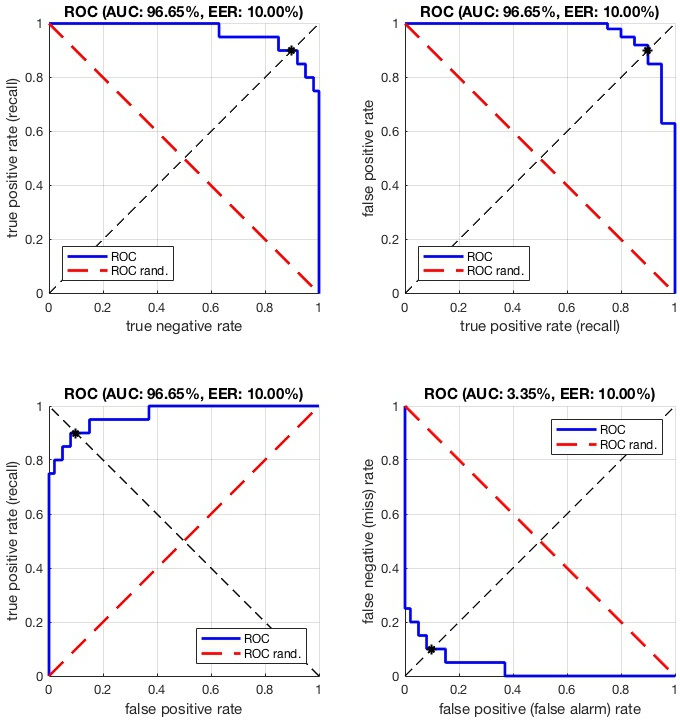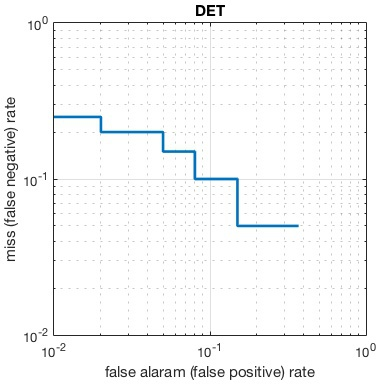This tutorial illustrates the use of the functions vl_roc, vl_det, and vl_pr to generate ROC, DET, and precision-recall curves.

VLFeat includes support for plotting starndard information retrieval curves such as the Receiver Operating Characteristic (ROC) and the Precision-Recall (PR) curves.

Consider a set of samples with labels labels and score scores. scores is typically the output of a classifier, with higher scores corresponding to positive labels. Ideally, sorting the data by decreasing scores should leave all the positive samples first and the negative samples last. In practice, a classifier is not perfect and the ranking is not ideal. The tools discussed in this tutorial allow to evaluate and visualize the quality of the ranking.

For the sake of the illustration generate some data randomly as follows:

numPos = 20 ;
numNeg = 100 ;
labels = [ones(1, numPos) -ones(1,numNeg)] ;
scores = randn(size(labels)) + labels ;


In this case, there have five times more negative samples than positive ones. The scores are correlated to the labels as expected, but do not allow for a perfect separation of the two classes.

# ROC and DET curves

To visualize the quality of the ranking, one can plot the ROC curve by using the vl_roc function:

vl_roc(labels, scores) ;


This produces the figureAn example ROC curve.

The ROC curve is the parametric curve given by the true positve rate (TPR) against the true negative rate (TNR). These two quantities can be obtained from vl_roc as follows:

[tpr, tnr] = vl_roc(labels, scores) ;


The TPR value tpr(k) is the percentage of positive samples that have rank smaller or equal than k (where ranks are assigned by decreasing scores). tnr(k) is instead the percentage of negative samples that have rank larger than k. Therefore, if one classifies the samples with rank smaller or equal than k to be positive and the rest to be negative, tpr(k) and tnr(k) are repsectively the probability that a positive/negative sample is classified correctly.

Moving from rank k to rank k+1, if the sample of rank k+1 is positive then tpr increases; otherwise tnr decreases. An ideal classifier has all the positive samples first, and the corresponding ROC curve is one that describes two sides of the unit square.

The Area Under the Curve (AUC) is an indicator of the overall quality of a ROC curve. For example, the ROC of the ideal classifier has AUC equal to 1. Another indicator is the Equal Error Rate (EER), the point on the ROC curve that corresponds to have an equal probability of miss-classifying a positive or negative sample. This point is obtained by intersecting the ROC curve with a diagonal of the unit square. Both AUC and EER can be computed by vl_roc:

[tpr, tnr, info] = vl_roc(labels, scores) ;
disp(info.auc) ;
disp(info.eer) ;


vl_roc has a couple of useful functionalities:

• Any sample with label equal to zero is effecitvely ignored in the evaluation.
• Samples with scores equal to -inf are assumed to be never retrieved by the classifier. For these, the TNR is conventionally set to be equal to zero.
• Additional negative and positive samples with -inf score can be added to the evaluation by means of the numNegatives and numPositives options. For example, vl_roc(labels,scores,'numNegatives',1e4) sets the number of negative samples to 10,000. This can be useful when evaluating large retrieval systems, for which one may want to record in labels and scores only the top ranked results from a classifier.
• Different variants of the ROC plot can be produced. For example vl_roc(labels,scores,'plot','tptn') swaps the two axis, plotting the TNR against the TPR. Since the TPR is also the recall (i.e., the percentage of positive samples retrieved up to a certain rank), this makes the plot more directly comparable to a precision-recall plot.Variants of the ROC plot.

A limitation of the ROC curves in evaluating a typical retrieval system is that they put equal emphasis on false positive and false negative errors. In a tipical retrieval application, however, the vast majority of the samples are negative, so the false negative rate is typically very small for any operating point of interest. Therefore the emphasis is usually on the very first portion of the rank, where the few positive samples should concentrate. This can be emphasized by using either precision-recall plot or a variant of the ROC curves called Detection Error Tradeoff (DET) curves.

A DET curve plots the FNR (also called false alarm rate) against teh FPR (also called miss rate) in logarithmic coordiantes. It can be generated by vl_det function call:An example DET curve.

# Precision-recall curves

Both ROC and DET curves normalize out the relative proportions of positive and negative samples. By contrast, a Precision-Recall (PR) curve reflects this directly. One can plot the PR curve by using the vl_pr function:

vl_pr(labels, scores) ;


This produces the figureAn example precision-recall curve.

The PR curve is the parametric curve given by precision and recall. These two quantities can be obtained from vl_roc as follows:

[recall, precision] = vl_roc(labels, scores) ;


The precision value precision(k) is the proportion of samples with rank smaller or equal than k-1 that are positive(where ranks are assigned by decreasing scores). recall(k) is instead the percentage of positive samples that have rank smaller or equal than k-1. For example, if the first two samples are one positive and one negative, precision(3) is 1/2. If there are in total 5 positive samples, then recall(3) is 1/5.

Moving from rank k to rank k+1, if the sample of rank k+1 is positive then both precision and recall increase; otherwise precision decreases and recall stays constant. This gives the PR curve a characteristic saw-shape. For aan ideal classifier that ranks all the positive samples first the PR curve is one that describes two sides of the unit square.

Similar to the ROC curves, the Area Under the Curve (AUC) can be used to summarize the quality of a ranking in term of precision and recall. This can be obtained as info.auc by

[rc, pr, info] = vl_pr(labels, scores) ;
disp(info.auc) ;
disp(info.ap) ;
disp(info.ap_interp_11) ;


The AUC is obtained by trapezoidal interpolation of the precision. An alternative and usually almost equivalent metric is the Average Precision (AP), returned as info.ap. This is the average of the precision obtained every time a new positive sample is recalled. It is the same as the AUC if precision is interpolated by constant segments and is the definition used by TREC most often. Finally, the 11 points interpolated average precision, returned as info.ap_interp_11. This is an older TREC definition and is obtained by taking the average of eleven precision values, obtained as the maximum precision for recalls largerer than 0.0, 0.1, ..., 1.0. This particular metric was used, for example, in the PASCAL VOC challenge until the 2008 edition.